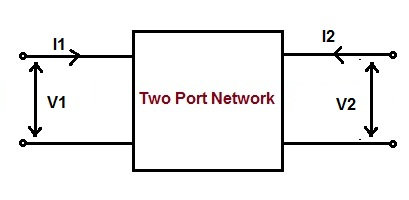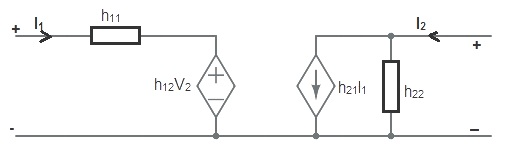# h Parameter or Hybrid Parameter of Two Port Network

h parameter of two port network is a square matrix of order 2×2. Basically it is a way to represent a two port network. It is also known as hybrid parameter. In this form of representation, voltage of input port and current of the output port is expressed in terms of current of input port and voltage of output port.

Thus, the h parameter for a two port network is defined as⇒V1 = h11I1 + h12V2……………(2)

⇒I2 = h21I1 + h22V2……………..(3)

### Why h Parameter is called Hybrid Parameter?

h parameter is also called hybrid parameter. This is because of the method of calculation of individual element of h matrix. Short circuit condition of input port is assumed for the calculation of h11 and h21. While open circuit condition is assumed for h12 and h22 calculation. Thus we see that, both open circuit and short circuit terminal conditions are assumed in the calculation of h11, h12, h21 and h22. This is the reason, it is also called hybrid parameter.

### Calculation of h Parameter:

Let us consider a two port network. Let V1, I1, V2 and I2 are the input voltage, input current, output voltage and output current respectively.Assuming the short circuit condition at the output terminal, we get

V2 = 0

Now putting V2 = 0 in (2), we get

V1 = h11I1

h11 = (V1 / I1)

Similarly putting V2 = 0 in (3), we get

I2 = h21I1

h21 = (I2 / I1)

Again assuming input port of the two port network to be open circuited, the input voltage will be zero.

I1 = 0

Now putting I1 = 0 in (2), we get

V1 = h12V2

h12 = (V1 / V2)

Similarly putting I1 = 0 in (3), we get

I2 = h22V2

h22 = (I2 / V2)

Thus there are four h parameter for a two port or four terminal network. Their values are tabulated below.

 h11 (V1 / I1) Condition: Output port of the two port network is short circuited i.e. V2 = 0 h21 (I2 / I1) h12 (V1 / V2) Condition: Input port of the two port network is open circuited i.e. I1 = 0 h22 (I2 / V2)

### Significance of Different Y Parameter:

• Since h11 is the ratio of input voltage and input current when the output port is short circuited, therefore it is known Input Impedance. Its unit is ohm.
• h22 is the ratio of output current and output voltage when input port is open circuited, therefore it is called Output Admittance of the network. Its unit is mho.
• h12 is the ratio of input voltage and output voltage when input port is open circuited, therefore it is called Reverse Voltage Gain. It is a unit less quantity.
• h21 is the ratio of output current and input current when output port is short circuited, therefore it is called Forward Current Gain. It is a unit less quantity.

### Equivalent Circuit Representation of h Parameter:

The equivalent circuit of h parameter for two port network can be represented using (2) and (3) as shown below.### 2 thoughts on “h Parameter or Hybrid Parameter of Two Port Network”

1.Shruti Sharma says:

This was awesome! Now I got command in all the three parameters ( h, y & z) ! These contents were so useful! Keep posting more. 🙂

1.admin says:

Thank you Shruti!

This site uses Akismet to reduce spam. Learn how your comment data is processed.